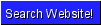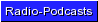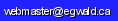Egwald Economics: Microeconomics

Cost Functions

by

Elmer G. Wiens

Egwald's popular web pages are provided without cost to users.
Please show your support by joining Egwald Web Services as a Facebook Fan:Follow Elmer Wiens on Twitter:Duality: Production / Cost Functions:   Cobb-Douglas Duality | CES Duality | Theory of Duality | Translog Duality - CES | Translog Duality - Generalized CES

I. The Cobb-Douglas Cost Function

If the underlying technology of production is Cobb-Douglas, it may be convenient to estimate the Cobb-Douglas parameters by way of the cost function, instead of directly using the Cobb-Douglas production function: Estimating a Cobb-Douglas production function.

The three factor Cobb-Douglas production function is:

q = A * (L^alpha) * (K^beta) * (M^gamma) = f(L,K,M).

where the quantities of inputs and output are:

L = labour, K = capital, M = materials and supplies, and q = product,

and, nu = (alpha + beta + gamma) is a measure of the returns of scale: decreasing returns to scale if nu < 1, constant returns to scale if nu = 1, and increasing returns to scale if nu > 1.

If we have a data set relating these inputs to output for varying levels of of inputs and output, we can estimate the parameters — A, alpha, beta and gamma — of the Cobb-Douglas production function directly.

Suppose, however, we have a data set relating the input (factor) prices, wL, wK, and wM, to the total cost of producing output for varying levels output, but do not have accurate data on the required levels of inputs. Then, we can work with the Cobb-Douglas cost function to estimate the parameters — A, alpha, beta and gamma.

The three factor Cobb-Douglas (total) cost function is:

C(q;wL,wK,wM) = h(q) * c(wL,wK,wM)

where the returns to scale function is:

h(q) = q^(1/(alpha+beta+gamma))

a continuous, increasing function of q (q >= 1), with h(0) = 0 and h(1) = 1.

and the unit cost function is:

c(wL,wK,wM) = B * [ wL^alpha * wK^beta * wM^gamma] ^(1/(alpha+beta+gamma))

with B = (alpha + beta + gamma) / [A * alpha^alpha * beta^beta * gamma^gamma] ^(1/(alpha+beta+gamma))

Note: while we do not know the values of A, alpha, beta, and gamma, we do know that B, and nu = (alpha + beta + gamma) are constants.

Rewrite the cost function by raising it to the power of (alpha + beta + gamma):

C^(alpha+beta+gamma) = c ^(alpha+beta+gamma) * h ^(alpha+beta+gamma) , or

C^(alpha+beta+gamma) = B ^(alpha+beta+gamma) * ([ wL^alpha * wK^beta * wM^gamma] ^(1/(alpha+beta+gamma)))^(alpha+beta+gamma) * (q^(1/(alpha+beta+gamma)))^(alpha+beta+gamma)

Letting B1 = B ^(alpha+beta+gamma), simplifying the expression, and introducing a parameter, delta, we get:

C^(alpha+beta+gamma) = B1 * [ wL^alpha * wK^beta * wM^gamma] * q ^delta

The purpose of the parameter, delta = 1?, will become evident.

If we knew the value of nu = (alpha + beta + gamma), we would have:

tC = C^nu = B1 * [ wL^alpha * wK^beta * wM^gamma] * q ^delta

Taking natural logarithms (log = ln), we get the linear equation:

log (tC) = nu*log(C) = log (B1) + alpha*log(wL) + beta*log(wK) + gamma*log(wM) + delta*log(q)       (*)

The plan is to guess at values of nu, and to compute tC = C^nu. Then, using linear regression with the equation (*) on our data set, we will estimate log(B1), a constant or intercept term, and the parameters alpha, beta, gamma, and delta, expecting that delta = 1. Then, we will compute the parameter A of the production function from the expression for B, since we now know B1, alpha, beta, and gamma.

We can repeat this process with different values of nu, until we estimate an equation that we like.

If we guess a value of nu that is too high, we expect that the estimated value of delta will also be too high (exceed 1), and vice versa. That is, the estimated value of delta will be an increasing function of nu, approximately equal to 1 for the correct value of nu. In other words, we expect the parameters nu and delta to be positively correlated, in order to balance the left hand and right hand side of equation (*).

Furthermore, we hope that the guessed value of nu will equal the sum of the estimated values of alpha, beta, and gamma.

The values of the variables of the regression equation (*) are generated by the web page's programs, and are displayed in the table, "Cobb-Douglas Cost Data Set." These variables' values are based on specific values of the parameters, A, alpha, beta, and gamma, which determine the underlying Cobb-Douglas technology.

The programs estimate the parameters of the regression equation (*) and display the results in the next table.
The default value of nu = 1.

QR Least Squares
Parameter Estimates
Parameter Coefficient std error t-ratio
log(B1)1.035165040866.167
alpha0.344568090641.424
beta0.453263068637.957
gamma0.202179086249.053
delta1.0869550607791.101
 R2 = 1 R2b = 1 # obs = 30

The estimated regression equation for (*) with nu = 1, and with the (rounded) estimated values of log(B1) = 1.0352, alpha = 0.3446, beta = 0.4533, gamma = 0.2022, and delta = 1.08696 becomes:

log (tC) = 1 * log(C) = 1.0352 + 0.3446 * log(wL) + 0.4533 * log(wK) + 0.2022 * log(wM) + 1.08696 * log(q)       (**)

The sum of the estimated parameters, alpha + beta + gamma = 0.3446 + 0.4533 + 0.186 = 1.0001 = nu1,
is a measure of the returns to scale of the underlying Cobb-Douglas technology.

For the "guessed at" value of nu = 1, the estimated value of delta = 1.08696 is greater than 1.

The value of nu is too large. Try a smaller value of nu.

 Cobb-Douglas Cost Function Parameter returns to scale: nu .5 < nu < 1.5

Change the value of the parameter "nu" and run a new regression using the same data set.

Since log(B1) = 1.0352, the estimated value of B1 = exp(1.0352) = 2.8157.
Since B1 = B ^(alpha+beta+gamma) = B^nu, B = B1^1/nu = B1^1/1.0001 = 2.8154.
Since B = (alpha + beta + gamma) / [A * alpha^alpha * beta^beta * gamma^gamma] ^(1/(alpha+beta+gamma)),
A = (((alpha+beta+gamma)/ B)^(alpha+beta+gamma)) / (alpha^alpha * beta^beta * gamma^gamma)
= ((1.0001/ 2.8154)^1.0001) / (0.3446^0.3446 * 0.4533^0.4533 * 0.2022^0.2022) = 1.014.

The estimated parameters of the exposed, underlying Cobb-Douglas technology are:
A = 1.014,   alpha = 0.3446, beta = 0.4533, and gamma = 0.2022.
Its Cobb-Douglas production function is:
f(L,K,M) = q = A * (L^alpha) * (K^beta) * (M^gamma)   =   1.014 * (L^0.3446) * (K^0.4533) * (M^0.2022)       (***).

Along with the estimated parameters of the production function, we have the Cobb-Douglas cost function
C(q;wL,wK,wM) = h(q) * c(wL,wK,wM)
= q^(delta/(alpha+beta+gamma)) * B * [ wL^alpha * wK^beta * wM^gamma] ^(1/(alpha+beta+gamma)), or
C(q;wL,wK,wM) = q^(1.08696/1.0001) * 2.8154 * [ wL^0.3446 * wK^0.4533 * wM^0.2022] ^(1/1.0001)       (****)

The Cobb-Douglas Cost Data Set table displays the data set values of the variables, wL, wK, wM, output (q), and total cost (C),
along with the estimated total cost (est C) using the equation (****).

 If we take the partial derivative of the cost function with respect to an input price, we get the estimated factor demand function for that input: ∂C/∂wL = L(q;wL,wK,wM) = h(q) * (alpha / wL) * c(wL,wK,wM) / (alpha + beta + gamma) = q^(1.08696/1.0001) * (0.3446 / wL) * c(wL,wK,wM) / (1.0001) ∂C/∂wK = K(q;wL,wK,wM) = h(q) * (beta / wK) * c(wL,wK,wM) / (alpha + beta + gamma) = q^(1.08696/1.0001) * (0.4533 / wK) * c(wL,wK,wM) / (1.0001) ∂C/∂wM = M(q;wL,wK,wM) = h(q) * (gamma / wM) * c(wL,wK,wM) / (alpha + beta + gamma) = q^(1.08696/1.0001) * (0.2022 / wM) * c(wL,wK,wM) / (1.0001)         The factor input quantities for each level of output are displayed in the table.

The factor shares for each input are obtained by:

 sL(q;wL,wK,wM) = wL * L(q;wL,wK,wM) / C(q;wL,wK,wM) = alpha / (alpha + beta + gamma), sK(q;wL,wK,wM) = wK * K(q;wL,wK,wM) / C(q;wL,wK,wM) = beta / (alpha + beta + gamma), sM(q;wL,wK,wM) = wM * M(q;wL,wK,wM) / C(q;wL,wK,wM) = gamma / (alpha + beta + gamma) and are independent of q, wL,wK, and wM.

The estimated factor demand elasticities are obtained by:

 εL,wL = ∂ln(L(q;wL,wK,wM))/∂ln(wL) = -(sK + sM), εL,wK = ∂ln(L(q;wL,wK,wM))/∂ln(wK) = sK, εL,wM = ∂ln(L(q;wL,wK,wM))/∂ln(wM) = sM, εL,q = ∂ln(L(q;wL,wK,wM))/∂ln(q) = delta / nu = 1.08696/1.0001, etc.
 εL,wL εL,wK εL,wM εL,q εK,wL εK,wK εK,wM εK,q εM,wL εM,wK εM,wM εM,q
 -0.6554 0.4533 0.2022 1.0869 0.3446 -0.5467 0.2022 1.0869 0.3446 0.4533 -0.7978 1.0869

To close the loop and to check our calculations, we also compute the level of output (est q) for the factor inputs using equation (***).

Generate a new data set by clicking on the "New Data Set" button, and thereby start from the beginning.

Cobb-Douglas Cost Data Set
—   Factor Prices   —     Output     — Total Cost —Factor InputsFactor Shares
Const  wL       wK       wM         q           C       est C  est qLKMsLsKsM
1 8.56 11.86  7.94   15  522.39    522.2      18.986     21.02    19.96   13.3    0.3446    0.4533   0.2022
1 7.84 12.34  4.78   16  499.53    499.34      20.363     21.95    18.34   21.12    0.3446    0.4532   0.2022
1 8.72 13.04  4.28   17  554.96    554.75      21.749     21.92    19.28   26.21    0.3446    0.4532   0.2022
1 8.6 12.32  6.56   18  624.43    624.19      23.143     25.01    22.96   19.24    0.3446    0.4532   0.2022
1 7.72 12.62  5.24   19  616.4    616.16      24.544     27.5    22.13   23.77    0.3446    0.4533   0.2021
1 6.98 12.76  4.58   20  615.67    615.43      25.952     30.38    21.86   27.17    0.3446    0.4532   0.2022
1 6.98 14.9  4.44   21  692.11    691.83      27.367     34.15    21.05   31.5    0.3445    0.4534   0.2022
1 7.34 14.74  7.26   22  814.16    813.83      28.785     38.2    25.03   22.66    0.3445    0.4533   0.2021
1 5.5 14.5  4.28   23  690.05    689.76      30.208     43.21    21.56   32.58    0.3445    0.4532   0.2022
1 6.78 15  5.12   24  817.88    817.54      31.638     41.55    24.7   32.28    0.3446    0.4532   0.2022
1 6.7 13.98  4.44   25  801.32    800.98      33.075     41.19    25.97   36.47    0.3445    0.4533   0.2022
1 7.04 12.48  6.08   26  860.97    860.61      34.519     42.12    31.26   28.62    0.3446    0.4533   0.2022
1 8.88 14.08  4.02   27  943.99    943.59      35.964     36.61    30.38   47.46    0.3445    0.4533   0.2022
1 6.8 12.92  7.28   28  971.44    971.02      37.414     49.2    34.07   26.97    0.3445    0.4533   0.2022
1 5.68 13.04  4.18   29  851.44    851.07      38.865     51.63    29.58   41.16    0.3446    0.4532   0.2022
1 8.94 12.9  5.82   30  1098.94    1098.45      40.329     42.34    38.6   38.16    0.3446    0.4533   0.2022
1 5.04 14.52  7.98   31  1051.21    1050.74      41.789     71.84    32.8   26.62    0.3446    0.4533   0.2022
1 8.1 11.86  6.22   32  1111.64    1111.14      43.255     47.27    42.46   36.12    0.3446    0.4532   0.2022
1 5.98 14.12  7.86   33  1174.8    1174.27      44.726     67.66    37.69   30.21    0.3446    0.4532   0.2022
1 6.2 14.8  7.28   34  1235.92    1235.36      46.202     68.66    37.83   34.31    0.3446    0.4532   0.2022
1 6.88 14.26  6.44   35  1268.13    1267.56      47.68     63.48    40.29   39.79    0.3446    0.4533   0.2022
1 7.54 11.1  6.5   36  1206.9    1206.35      49.164     55.13    49.26   37.52    0.3446    0.4533   0.2022
1 5.02 13.74  6.12   37  1176.1    1175.56      50.652     80.69    38.78   38.84    0.3446    0.4533   0.2022
1 7.18 14.22  4.94   38  1332.1    1331.48      52.14     63.9    42.44   54.49    0.3446    0.4533   0.2022
1 6.68 11.3  5.04   39  1209.24    1208.68      53.634     62.35    48.48   48.49    0.3446    0.4532   0.2022
1 8.08 11.22  4.18   40  1273.83    1273.23      55.128     54.3    51.43   61.58    0.3446    0.4532   0.2022
1 6 13.44  7.94   41  1459.16    1458.47      56.633     83.76    49.19   37.14    0.3446    0.4533   0.2022
1 8.38 12.22  5.22   42  1478.82    1478.11      58.131     60.78    54.82   57.25    0.3446    0.4532   0.2022
1 5.42 12.58  6.48   43  1382.02    1381.36      59.639     87.82    49.77   43.1    0.3446    0.4533   0.2022
1 8.5 11.48  4.44   44  1470.6    1469.89      61.146     59.59    58.03   66.93    0.3446    0.4532   0.2022

The observations in the data set on this web page are generated without an error variable. If the guessed value of nu, the measure of the returns to scale, is incorrect, then the estimates of A, alpha, beta and gamma are incorrect and the Cobb-Douglas production function does not produce the right output or q value in the above table. However, the incorrect estimates of A, alpha, beta and gamma will produce estimates of the costs for each level of output, q, which agree with the actual costs.Copyright © Elmer G. Wiens:   Egwald Web ServicesAll Rights Reserved.    Inquiries